# Subtracting DecimalsExample 1: A customer buys \$6.33 of food in a store. If he pays with a \$10 bill, then how much change should the cashier give him?

Analysis: The cashier needs to subtract the two decimals in order to make change for the customer.

Step 1: You must first line up the decimal points in a column.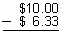Step 2: Start on the right, and subtract each column in turn. Note that you are subtracting digits in the same place-value position.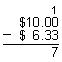Step 3: If the digit you are subtracting is bigger than the digit you are subtracting from, you have to borrow a group of ten from the column to the left.Step 4: Be sure to place the decimal point in the difference.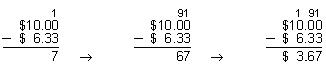Answer: The cashier should give the customer \$3.67 in change.

Example 2: Subtract: 8.06 - 8.019

Step 1: You must first line up the decimal points in a column.Step 2: The decimals in this problem do not have the same number of decimal digits. You can write an extra zero to the right of the last digit of the first decimal so that both decimals have the same number of decimal digits.Step 3: Start on the right, and subtract each column in turn. (Subtract digits in the same place-value position.)Step 4: If the digit you are subtracting is bigger than the digit you are subtracting from, you have to borrow a group of ten from the column to the left.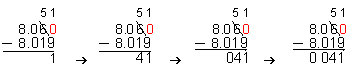Step 5: Be sure to place the decimal point in the difference.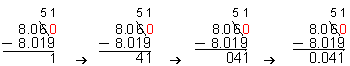In Example 1, we used four steps to subtract decimals. However, in Example 2, we had the extra step of writing an extra zero to the right of the last digit of the first decimal so that both decimals have the same number of decimal digits. Accordingly, we have summarized the 5-step procedure for subtracting decimals below.Procedure: To subtract decimals, follow these steps:

1. Line up the decimal points in a column.
2. When needed, write one or more extra zeros to the right so that both decimals have the same number of decimal digits.
3. Start on the right, and subtract each column in turn. (Subtract digits in the same place-value position.)
4. If the digit you are subtracting is bigger than the digit you are subtracting from, you have to borrow a group of ten from the column to the left.
5. Place the decimal point in the difference.Example 3: Two students were asked to subtract these numbers: \$88 - \$77.23. Student 1 calculated a difference of \$11.23 and Student 2 calculated a difference of \$10.77. Which student is correct? Explain your answer.

Student 1: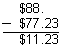Student 2:Analysis: Student 1 did not follow the procedure for subtracting decimals: He did not write extra zeros as place holders and he did not borrow. As a result, the difference calculated by Student 1 is incorrect. Student 2 followed the correct procedure and calculated the correct difference.

Answer: Student 2 is correct: \$88 - \$77.23 = \$10.77

Example 4: Subtract: 32.5 - 7.94Answer: 32.5 - 7.94 = 24.56

Example 5: Subtract: 30.041 - 9.785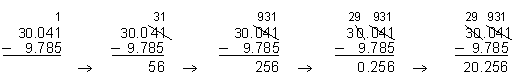Answer: 30.041 - 9.785 = 20.256

Example 6: Subtract: 18.2 - 3.199Answer: 18.2 - 3.199 = 15.001Example 7: In a 200-meter dash, the first-place winner reached the finish line in 19.75 s and the second-place winner reached the finish line in 19.8 s. What is the difference in their times?

Answer: The difference in their times is 0.05 s.

Summary: When subtracting decimals, first line up all the decimal points in a column. When needed, write one or more extra zeros to the right so that both decimals have the same number of decimal digits. Subtract digits in the same place-value position. When needed, borrow a group of ten from the column to the left. Place the decimal point in the difference.

### Exercises

Directions: Read each question below. You may use paper and pencil to help you subtract. Click once in an ANSWER BOX and type in your answer; then click ENTER. After you click ENTER, a message will appear in the RESULTS BOX to indicate whether your answer is correct or incorrect. To start over, click CLEAR.

 1 Subtract:  27.098 - 6.5 ANSWER BOX:   RESULTS BOX:
 2 Subtract: \$329 - \$76.89 ANSWER BOX: \$  RESULTS BOX:
 3 Subtract: 84.3 - 0.863 ANSWER BOX:   RESULTS BOX:
 4 Subtract: 45.059 - 19.724 ANSWER BOX:   RESULTS BOX:
 5 Professional football games have four 15-minute quarters. If a game totals 37.5 minutes so far, how much time is left in the game? ANSWER BOX:  min  RESULTS BOX: